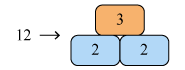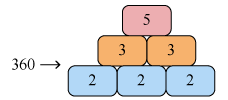# The Fundamental Theorem of Arithmetic

Go back to  'Arithmetic-Integers'

### What is the Fundamental Theorem Of Arithmetic?

The Fundamental Theorem Of Arithmetic says that every composite number can be factorized as a product of primes, and this factorization is unique, apart from the order in which the prime factors occur. For example, consider any number, say 240. Our theorem tells us that this must be expressible as a product of primes. Below, we have factorized this number into a product of primes:

$\begin{array}{l}240 = 2 \times 2 \times 2 \times 2 \times 3 \times 5\\\;\;\;\;\;\; \;= {2^4} \times {3^1} \times {5^1}\end{array}$

Our theorem further tells us that this factorization must be unique. That is, there will exist no other way to express 240 as a product of primes. Of course, we can change the order in which the prime factors occur. For example, the prime factorization can be written as:

$\begin{array}{l}240 = {3^1} \times {2^4} \times {5^1}\\\;\;\;\;\;\,\;\, = {3^1} \times {2^2} \times {5^1} \times {2^2}\,{\rm{etc}}{\rm{.}}\end{array}$

But the set of prime factors (and the number of times each factor occurs) is unique. That is, 240 can have only one possible prime factorization, with four factors of 2, one factor of 3, and one factor of 5.

### Visually understanding the Fundamental Theorem Of Arithmetic

The best way to understand the Fundamental Theorem Of Arithmetic is to think of prime numbers as the building blocks, or "bricks", of the system of natural numbers. Every natural number can be built using primes. Let's take a couple of examples to understand this better.

Example. $$12 = 2 \times 2 \times 3$$, so 12 can be built using two bricks of the prime number 2, and one brick of the prime number 3, as shown below:This is the only way to build the number 12. No other combination of prime bricks can form 12. Convince yourself about this fact.

Example. $$360 = 2 \times 2 \times 2 \times 3 \times 3 \times 5$$, so 360 can be built using three bricks of the prime number 2, two bricks of the prime number 3, and one brick of the prime number 5, as shown below:Once again, this combination of prime bricks is the only way to build the number 360.

Summary of the key idea. So what the Fundamental Theorem Of Arithmetic really says is that every number has a unique brick structure - every number is made up of a unique combination of prime bricks. This is the best way to understand this theorem.

### Writing the prime-factorised form of a number

Generally, we will express the prime factors of a number in ascending order. Also, you are already familiar with how to determine the set of prime factors of a number. Basically, we try to determine prime divisors of that number, starting from the lowest possible prime factor. Let us take an example, and determine the prime factorization of 24000:

$\begin{array}{*{20}{l}} 2\vert {24000}\\\hline2\vert {12000}\\\hline2\vert {6000}\\\hline2\vert {3000}\\\hline2\vert {1500}\\\hline2\vert {750}\\\hline3\vert {375}\\\hline5\vert {125}\\\hline5\vert {25}\\\hline5\vert 5\\\hline{\;\;}\vert 1\end{array}$

Thus, the prime factorization of 24000 is:

$24000 = {2^6} \times {3^1} \times {5^3}$

According to the Fundamental Theorem Of Arithmetic, this factorization is unique, that is, 24000 can be expressed as a product of primes in no other way (except of course for the order of the prime factors).

### Examples on the Fundamental Theorem Of Arithmetic

Example-1. A composite number x is prime factorized as follows:

$x = {p^a}{q^b}$

If the last digit of x is 0, what can we say about the primes p and q?

Solution. The last digit of any number can be 0 only if it is a multiple of 10. The prime factors of 10 are 2 and 5, that is, 10 = 2 × 5. Thus, 2 and 5 must be factors of x as well. Based on this observation and the Fundamental Theorem Of Arithmetic, we conclude that one of p and q must be 2, and the other must be 5. Only then can x be a multiple of 10.

Example-2. Determine the prime factorization of 21660.

Solution. We have:

$\begin{array}{*{20}{l}}\;2\vert {21660}\\\hline\;2\vert {10830}\\\hline\;3\vert {5415}\\\hline\;5\vert {1805}\\\hline{\!\!19}\vert {361}\\\hline{\!\!19}\vert {19}\\\hline{\;\;\;}\vert 1\\\\\end{array}$

Thus, the required prime factorization is:

$21660 = {2^2} \times {3^1} \times {5^1} \times {19^2}$

Example-3. Determine the prime factorization of 1310400.

Solution. We have:

$\begin{array}{*{20}{l}}\;\,2 \vert {1310400}\\\hline\;\,2 \vert {655200}\\\hline\;\,2 \vert {327600}\\\hline\;\,2 \vert {163800}\\\hline\;\,2 \vert {81900}\\\hline\;\,2 \vert {40950}\\\hline\;\,3 \vert {20475}\\\hline\;\,3 \vert {6825}\\\hline\;\,5 \vert {2275}\\\hline\;\,5 \vert {455}\\\hline\;\,7 \vert {91}\\\hline{13} \vert {13}\\\hline{\;\;\;\,} \vert 1\end{array}$ Thus, the required prime factorization is:

$1310400 = {2^6} \times {3^2} \times {5^2} \times {7^1} \times {13^1}$

Learn math from the experts and clarify doubts instantly

• Instant doubt clearing (live one on one)
• Learn from India’s best math teachers
• Completely personalized curriculum